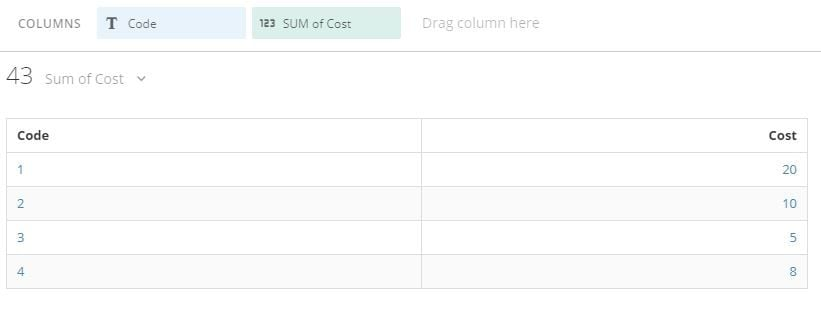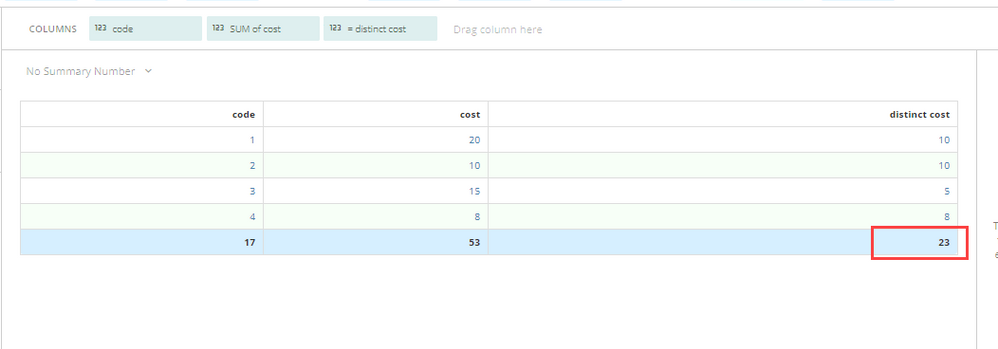# sum based on distinct in another column

hello, this seems to be straight forward, but I could not get it.

I wanted to sum up the cost based on distinct code, but my beast mode does nto work:

a sample data, I wanted to calculate sum of cost by distinct code, the sum should be 33.  each code has the same cost.

this is my beast mode:

SUM(
CASE
WHEN(distinct `code') then `cost`
END
)

 code cost 1 10 2 10 3 5 1 10 4 8

• I added an indicator in the dataflow.  Every code when it is the first time presneted in the data, the indicator is 1, then I sumed up based on this indicator.

sum(
case
when `code_indicator` = 1 then `mc_spend`
else 0
end
)

•ContributorIs something stopping you from just displaying the SUM of Cost by Code on a chart since normally in Domo if you were to put Code and then SUM of Cost on one card it would already do what you're asking without a beastmode.**Make sure to like any users posts that helped you and accept the ones who solved your issue.**
• As you can see sum in the table has code 1 sumed up as 20, so I will get total of 43.  But the actual sum is 33, in other words, I only want to sum for each code once.

in adition, after I get the sum, I will do some other calculations such  as cost per visit, cost per download etc.

•CoachThis isn't something that you can do within a beastmode calculation.  Especially if you are going to perform further calculations on it.  I would recommend creating a new data set where you agregate this data at different levels.  (once aggregated by visit id, once by download id, etc.)

You could use

`SUM(DISTINCT `Cost`)`

but that will only work to get the correct value for each row in the table, the Total row will still be off:“There is a superhero in all of us, we just need the courage to put on the cape.” -Superman
• sum (distinct 'cost' ) could not give me the correct sum either.  My dataset has 70m rows and 200 columns, and it is updated daily, I have many other calcualtions (combinations)  I would like to do.  I hope I can do it in beast mode instead of in dataflow.

• I added an indicator in the dataflow.  Every code when it is the first time presneted in the data, the indicator is 1, then I sumed up based on this indicator.

sum(
case
when `code_indicator` = 1 then `mc_spend`
else 0
end
)